# M7-S8: The Quantum Theory and Wien's Displacement Law#### Important terminologies and concepts

• Black body: an idealistic object that absorbs all radiation frequencies/wavelengths upon falling within its vicinity.

A black body at thermal equilibrium and given temperature would also emit radiation of exactly the same amount as what it first absorbed.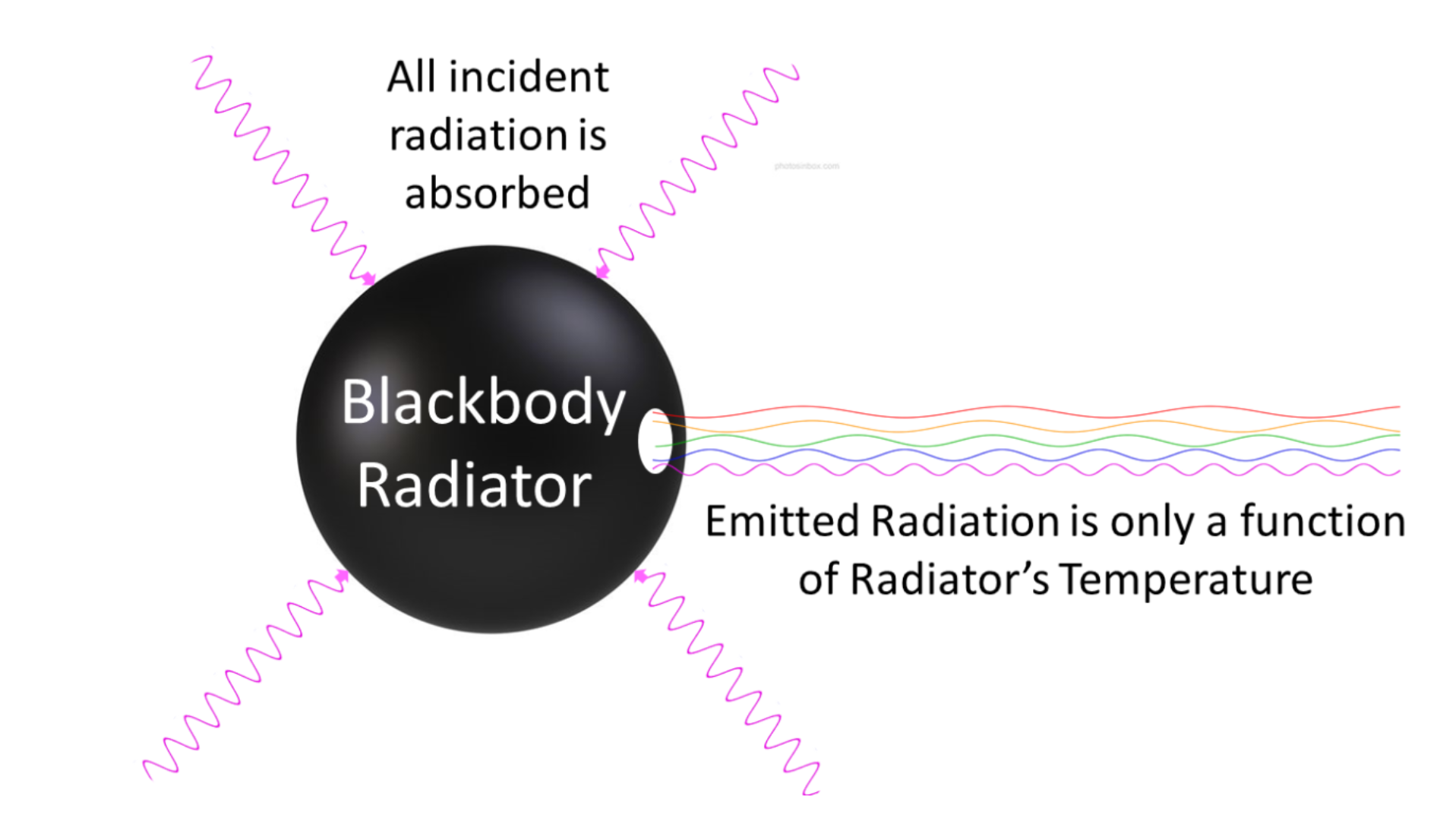Black body radiation must obey the second law of thermodynamics
The essential point is that if we suppose a particular body can absorb better than it emits, then in a room full of objects all at the same temperature, it will absorb radiation from the other bodies better than it radiates energy back to them. This means it will get hotter, and the rest of the room will grow colder, contradicting the second law of thermodynamics (entropy of a system always increases).

The converse cannot be true as it violates the first law of thermodynamics (conservation of energy).

Are black bodies real?
No, but scientists have been able to produce objects that possess similar properties as a black body.Figure showing the theoretical path of radiation after it enters a black body-like cavity.
A large box with a small hole is a simple example of a near-perfect black body. Once radiation enters the box via the opening, it bounces around in the box, unable to exit.

In 1895, at the University of Berlin, Wien and Lummer punched a small hole in the side of an otherwise completely closed oven. They began to measure the radiation coming out. The beam coming out of the hole was passed through a diffraction grating, which sent the different wavelengths/frequencies in different directions, all towards a screen. A detector was moved up and down along the screen to determine the intensity of each frequency of emitted radiation.

• Thermal equilibrium: a state where there is no net flow or transfer of heat energy between objects. In simple terms, the temperature of an object at thermal equilibrium remains constant.
• Classical Theory of Electromagnetic Radiation (Rayleigh-Jeans Law)
The classical theory states that the intensity of black body radiation is directly proportional to its frequency. That is, greater the frequency a radiation has, the more energy this is equivalent to. The theory always states that the radiation emitted by a black body is continuous and a function of temperature; greater a black body’s temperature, more energy it emits.
The development of Rayleigh-Jeans Law is based on many assumptions and knowledge in physics at the time. Rayleigh and Jeans assumed radiation would also be continuous, similar to other physical quantities such as velocity and acceleration.

#### Problem – Ultraviolet Catastrophe

By this logic, the classical model proposes that a black body at thermal equilibrium would emit radiation at all frequencies, resulting in an infinite amount of energy. However, this disobeys the Law of Conservation of Energy as it is merely impossible to emit ‘infinite’ amount of energy.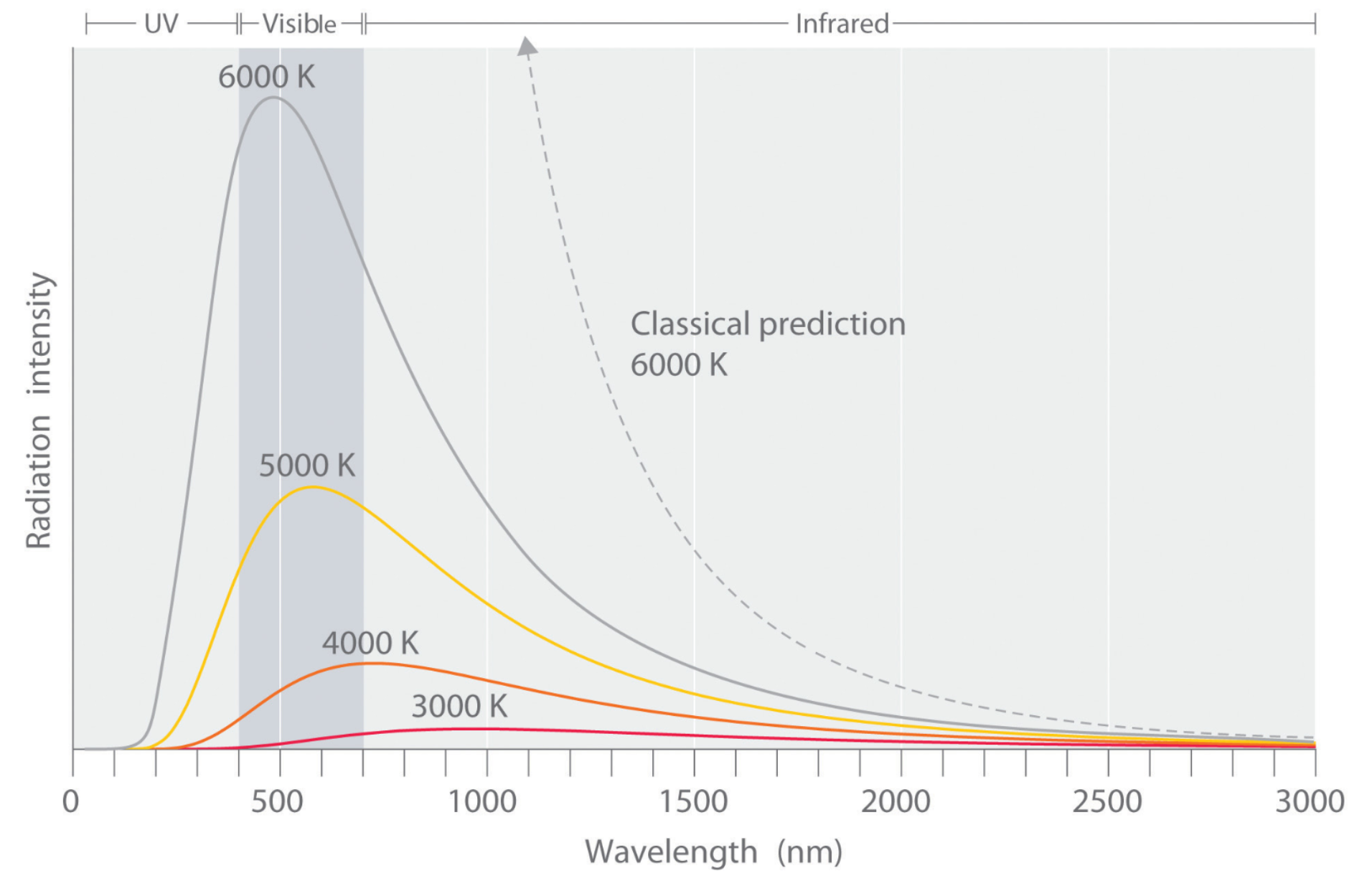Figure showing the ultraviolet catastrophe - inconsistent predications of classical and quantum theories of physics with regard to energy emitted by a black body radiation.

Experimentally, the classical theory of radiation is only valid to an extent. For radiation with frequencies below 105 GHz (1017 Hz), the energy measured is consistent with the model.

However, as the frequency becomes higher, the inconsistency between the classical theory and experimental results grows larger and larger. The divergent trend becomes most evident in the ultraviolet spectrum. This observation gave rise to the ultraviolet catastrophe.

The ultraviolet catastrophe is also called Rayleigh-Jeans catastrophe.

#### Planck’s quantum theory resolved the ultraviolet catastrophe

• Max Planck explained the ultraviolet catastrophe by stating that energy absorbed and emitted by a black body is quantised instead of being continuous.
• Quantisation refers to the division of an object, entity or measurement into its smallest units. When something is quantised, it means it consists of a multiple of smaller units of the same thing.
• For example, the Australian currency is quantised as any amount of money can be divided into a finite number of 5-cent coins. In this case, 5-cent coins are the currency's smallest units.
• However, when Planck proposed the concept of quantisation, he was specifically referring to energy.
• Planck proposed that energy is gained and lost cannot be any random value but must be in integral multiples of the smallest unit of energy - a quantum.
• Note: a photon is a quantum for electromagnetic radiation but the name itself was later proposed by Einstein, not Planck. In this context, 'quantum' and 'photon' are interchangeable.

The energy of a photon (quantum) is given by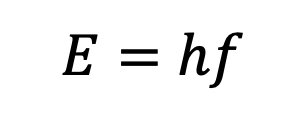The energy of total radiation emitted is therefore given by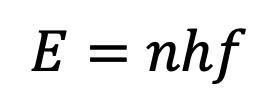Where h is Planck’s constant: 6.626 × 10-34 Js (Joule second); n is an integer (now called a quantum number)

• Since the energy contained within a photon (quantum) is finite, it means that energy emitted by a black body is also finite (rather than infinite as proposed by the classical theory). In particular, depending on a black body’s temperature, the intensity distribution of photons of various frequencies is different. See diagram above for reference.

Practice Question 1
(a) Calculate the energy of a photon with frequency 2 × 108 (1 mark)

(b) The radiation in (a) has an intensity of 100 Wm-2, how many photons are striking a 5 m2 area per second? (1 mark) Recall that a Watt equals to Joules/second

#### Wilhelm Wien’s Law builds upon Planck’s quantisation theory

• Wien’s displacement law states that the black-body radiation curve for different temperatures will peak at different frequencies/wavelengths. In specific, the wavelength that produces the highest intensity varies inversely with temperature; higher the temperature, shorter the wavelength.

Wien’s Law is given by: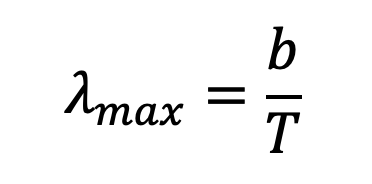Where b is Wien’s displacement constant: 2.898 × 10-3 metre Kelvin (mK); and T is the object’s temperature in Kelvins (K). The wavelength derived from this equation would have units in metres (m).

Examples of Wien’s Law

• Stellar emission. Some stars radiate partially in the visible spectrum. The intensity distribution of a star’s emission depends on its surface temperature.Hertzsprung-Russell diagram shows different groups of stars, each classified according to various characteristics such as temperature.

• Mammals with a body/skin temperature of around 300 K emit infrared radiation. This can be observed with infrared detectors:Mammals' skin temperature emits infrared radiation which can be captured by specialised detectors.

• Fire produced from burning wood has an average temperature of about 1500 K. This means majority of its radiation has a wavelength around 2000 nm.However, the reason why we see fire is because it also emits a small portion of visible light. This is also the reason why wood fire can provide a large amount of heat but is not efficient at providing lighting.

Practice Question 2

(a) The Sun has a surface temperature of 5700 K, what is the wavelength of maximum intensity of solar radiation?

(b) The maximum intensity radiation emitted from another star in the Milky Way has a wavelength of 1800 nm.

What is its surface temperature in degrees Celsius?

Previous section: Polarisation of Light

Next section: The Photoelectric Effect